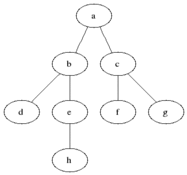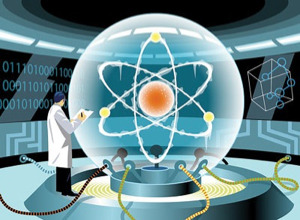# Windows on quantum computing

It is recent news that over the past decade Microsoft has been pursuing a research project aimed at building a quantum computer. The news may appear surprising, given that quantum computation is generally thought to be an object of far-fetched theoretical research. But is it really so? In this post we’ll get a taste of what quantum computation is, and how far (or how close) we may be to it.

#### What is a qbit

Where a classical computer has bits, a quantum computer has quantum bits or qbits. Classical bits have two mutually exclusive states: 0 or 1. Quantum bits, instead, being quantum systems, are in a superposition of the two states, meaning that they actually exist in both states simultaneously, each with a probability coefficient.
When n qbits are put together, the set of qbits stores simultaneously all 2^n possible combinations of 0 and 1, again each with a certain probability.Now this is the key to understanding why quantum computers may be exponentially faster than classical ones.
When a computer runs through an algorithm it has to descend sequentially all the nodes of each branch in the solution tree in order to identify the solution, as illustrated in the picture.
A quantum computer, instead, stores at every time step all the possible states and thus is able to visit stepwise all of the branches in parallel. In theoretical terms, the quantum computer behaves like a non-deterministic Turing machine.

#### Problems

Unfortunately, quantum computers are not just like non-deterministic Turing machines.As long as the set of quantum bits is left on its own, it effectively does keep stored all the possible states of the system; however, in order to get the output from the computer, it is necessary to perform a measure upon the quantum bit. Now measure on a quantum system is tricky, since it effectively destroys the superposition of states. Before the measure the system was a mixture of all possible states, with various probability weights; after the measure, the system is identified to be in one specific state, just one among the many possibilities.

The fact that we collapse the system in one state means that we loose track of all the other branches that were visited and of all the other results. This information loss that happens when we try to read the output from a quantum computer is the real conceptual issue of quantum computing.

#### The challenge: quantum algorithms

Measures on a quantum state result in a loss of information, but the amount of information lost depends on the possible states of the system. This means that the computation performed by the quantum bits can be exploited in the most efficient manner through a ‘clever’ choice of the output states.

Think about picking out an item from a box which has been shaken (relax, there’s no cat inside). If the box contained just slips of paper of various colors, the probability of drawing out a white slip will be just average. On the other hand if the box contained just white slips of paper together with other, weightier objects, it is likely that the first object you’ll find on top of the box is a white slip of paper.
Efficient quantum algorithms are equivalent to this: smart arrangements of the box content (i.e. of the states contained in the superposition) that make the correct result most probable and thus easier to find.
This is the case of the famous algorithm by Shor which runs integer factorization in polynomial time.

The reason why we have yet so few quantum algorithms like Shor’s is that the principles of quantum computation are still largely far from understood.
Quantum programming is an exciting field, combining computer science and quantum mechanics; understanding the principles that make an efficient quantum algorithm may prove to be as important, in order to exploit the power of quantum computation, as building a working instance of a quantum computer – and very possibly more so.